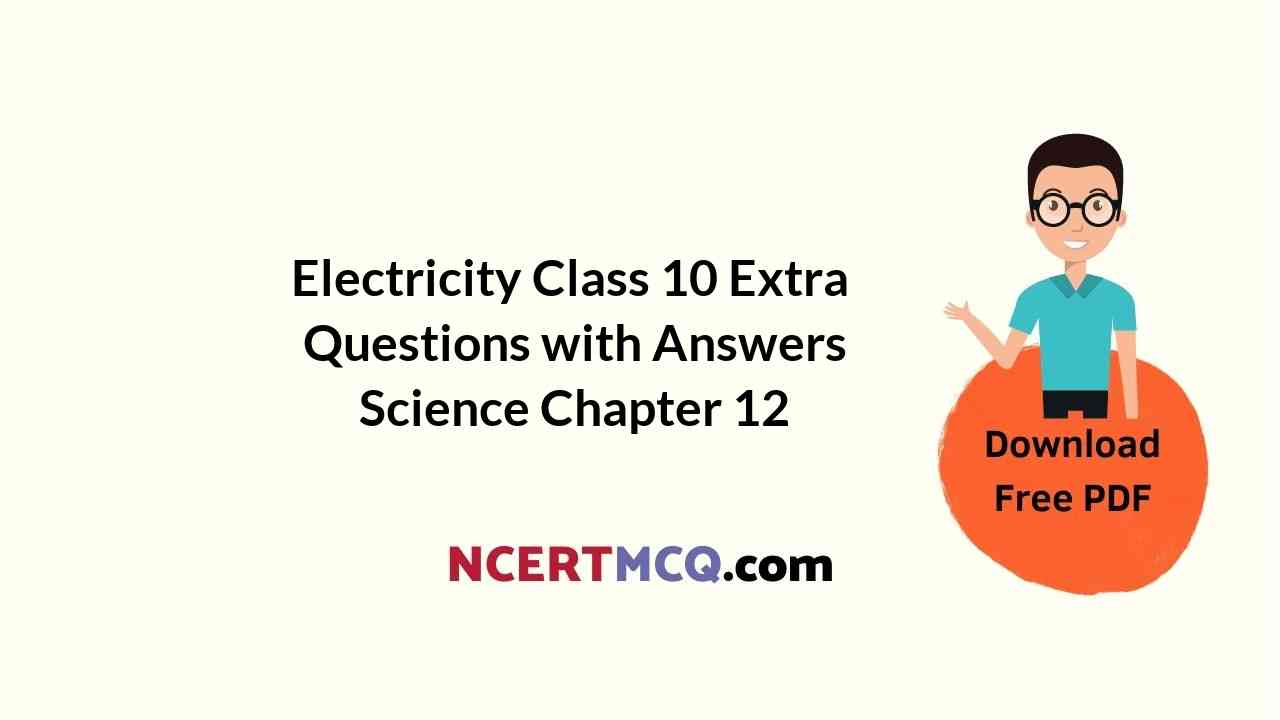In this page, we are providing Online Education Electricity Class 10 Extra Questions and Answers Science Chapter 12 pdf download. NCERT Extra Questions for Class 10 Science Chapter 12 Electricity with Answers will help to score more marks in your CBSE Board Exams. https://ncertmcq.com/extra-questions-for-class-10-science/

## Online Education for Class 10 Science Chapter 12 Extra Questions and Answers Electricity

Extra Questions for Class 10 Science Chapter 12 Electricity with Answers Solutions

### Extra Questions for Class 10 Science Chapter 12 Very Short Answer Type

Electricity Class 10 Extra Questions Question 1.
Define the following terms:
(a) potential difference
(b) 1 volt
(c) electric current
(d) one ampere.
Potential difference: The potential difference between two points in an electric circuit is the work done to move a unit charge from one point to the other. It is given by:One volt/volt: The SI unit of potential difference is volt (V). One volt is the potential difference between two points in an electric circuit when one joule of work is done to move a charge of one coulomb from one point to the other.

Electric current: Electric current is the rate of flow of electric charges. It is denoted by I. It is given by: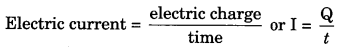One ampere/ampere: The SI unit of electric current is ampere (A). One ampere is the electric current when one coulomb of charge flows through a conductor in one second.

Class 10 Electricity Extra Questions Question 2.
What are resistors? Give some examples.
Substances having comparatively high electrical resistance are called resistors. Some examples of resistors are alloys like nichrome, manganin, constantan, etc.

Extra Questions Of Electricity Class 10 Question 3.
Keeping the potential difference constant, the resistance of a circuit is doubled. By how much does the current change?
V = IR
$$\frac{V}{I}$$ = R
Since the resistance and the current are inversely proportional, the current will become half.

How does the resistance of a wire change when:
(a) Its length is tripled?
(b) Its diameter is tripled?
(c) Its material is changed to one whose resistivity is three time?
(a) The resistance is tripled.
(b) The resistance is reduced by 9 times.
(c) The resistance is tripled.

Electricity Class 10 Extra Questions Numerical Question 5.
If a wire is increased to 4 times its original length, by what factor does the resistivity change?
Since the resistivity depends only on the material of the wire, and not its length, it will remain the same.

Electricity Extra Questions Class 10 Question 6.
If the current passing through a conductor is doubled, what will be the change in the heat produced?
Heat produced will increase by four times (H ∝ I2)

Extra Questions On Electricity Class 10 Question 7.
Name some devices which work on heating effect of electric current.

• Electric bulb
• Electric iron
• Electric geyser
• Electric fuse

Class 10 Science Chapter 12 Extra Questions Question 8.
Why is an electric bulb filled with argon and nitrogen gas?
An electric bulb is filled with argon and nitrogen gas because they do not react with the hot tungsten filament and hence, prolong the life of the filament of the electric bulb.

Electricity Class 10 Questions And Answers Question 9.
State the Joule’s law of heating.
Joule’s law of heating states that the heat produced in a resistor is directly proportional to

• Square of current (I2)
• Resistance of the resistor (R) and
• Time for which the current flows through the resistor.

H = I2Rt joules
From Ohm’s law, we get H = VIt joules = v2t/R joules

Class 10 Science Electricity Extra Questions Question 10.
How is heating effect of electric current used in an electric bulb?
Electric bulb works on the principle of heating effect of electric current. When electric current passes through a very thin, high resistance tungsten filament of an electric bulb, the filament becomes white hot and emits light.

Class 10 Physics Electricity Extra Questions Question 11.
Explain why, the filaments of electric bulbs are made of tungsten.
The filaments of electric bulbs are made of tungsten because it has a very high resistance. Due to its high resistance, heat produced is high and it becomes white-hot emitting light. Also due to its high melting point (3380° C), it can be kept white hot without melting.

Extra Questions For Electricity Class 10 Question 12.
How much work is done in moving a charge of magnitude 3 C across two points having a potential difference of 12 V?
Given : Q = 3 C, V = 12 V
To find: W
V = $$\frac{W}{Q}$$
W = VQ = 12 × 3 = 36 J

Extra Questions Electricity Class 10 Question 13.
A current of 4 A flows through a 12V car headlight bulb for 10 minutes. How much energy transfer occurs during this time?
Given : I = 4 A, V = 12 V, t = 10 min = 600 s
Energy transferred = VIt = 12 × 4 × 600 = 28800 J.

Chapter 12 Science Class 10 Extra Questions Question 14.
When a 12 V battery is connected across an unknown resistor, there is a current of 2.5 mA in the circuit. Find the value of the resistance of the resistor.
Here, V = 12 V, I = 2.5 mA = 2.5 × 10-3 A
Resistance,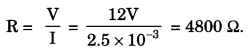Ch 12 Science Class 10 Extra Questions Question 15.
An electric current of 4.0 A flows through a 12 Ω resistor. What is the rate at which heat energy is produced in the resistor?
Given: I = 4 A, R = 12 Ω
Rate of production of heat energy, P = I2R = 42 × 12 = 192 W.

Question 16.
A heating element is marked 210 V, 630 W. What is the current drawn by the element when connected to a 210 V D.C. mains? What is the resistance of the element?
Given, P = 630 W, V = 210V
Current drawn, I = $$\frac{P}{V}=\frac{630}{210}$$ = 3A

Question 17.
Calculate the energy transferred by a 5 A current flowing through a resistor of 2 Ω for 30 minutes.
Here, I = 5 A, R = 2 Ω, t = 30 min = 1800 s
Energy transferred = I2Rt = (5)2 × 2 × 1800 = 9 × 104 J.

Question 18.
What does the slope of V – I graph at any point represent?
Resistance.

Question 19.
What is the shape of the graph obtained by plotting potential difference applied across a conductor against the current flowing through it?
Straight line.

Question 20.
Give reason why metals are good conductors, whereas non-metals are bad conductors of electricity.

• Metals contain free electrons which help in the conduction of electric charge and hence current
• Resistivity of metals is higher

Question 21.
The electrical resistivity of silver is 1.60 × 10-6 Ω m. What will be the resistance of a silver wire of length 10 m and cross-sectional area 2 × 10-3 m2?
Given: p = 1.60 × 10-6 Ω m, l = 10 m and A = 2 x 10-3 m2
Resistance,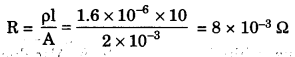Question 22.
Why are copper and aluminium wires usually employed for electricity transmission?
Copper and aluminium have low resistivities. When electricity is transmitted through copper and aluminium wires, the power losses in the form of heat are very small.

### Extra Questions for Class 10 Science Chapter 12 Short Answer Type I

Question 1.
Sketch a circuit diagram of an electric circuit consisting of a cell, an electric bulb, an ammeter, a voltmeter and a plug key.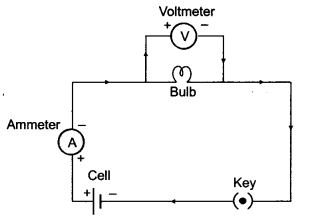Question 2.
Differentiate between Resistance and Resistivity.
Resistance:

1. It is the opposition provided by the atoms of a conductor to the flow of electrons.
2. SI unit of resistance is Ω (Ohm).
3. Resistance depends on length, area of cross section, material and temperature of conductor.

Resistivity:

1. It is the resistance of the conductor of that substance of unit length and unit area of cross section.
2. SI unit of Resistivity of Ωm (Ohm-meter).
3. Resistivity of substance depends only on the material of substance.

Question 3.
Distinguish between resistances in series and resistances in parallel.
Resistances in series:

1. If a number of resistances are connected in such a way that the same current flows through each resistance, then the arrangement is called resistances in series.
2. The current across each resistance is same.
3. The equivalent resistance in series combination is greater than the individual resistances.
4. This combination decreases the current.in the circuit.

Resistances in parallel:

1. If a number of resistances are connected between two common points in such a way that the potential differences across each of them is the same, then the arrangement is called resistances in parallel.
2. The voltage across each resistance is same.
3. The equivalent resistance in parallel combination is smaller than each of the individual resistances.
4. This combination increases the current in the circuit.

Question 4.
Nichrome wire is used for making the Ideating elements of electrical appliances like iron, geyser, etc. Give reasons.
Nichrome wire is used for making the heating elements of electrical appliances like iron, geyser, etc. because:

• Nichrome has a very high resistance due to which it produces a lot of heat on passing current.
• It does not undergo oxidation easily even at high temperature due to which it can be kept red hot.

Question 5.
A copper wire of resistivity 2.6 × 10-3 Ωm, has a cross sectional area of 30 × 10-4 cm3. Calculate the length of this wire required to make a 10 Ω coil.
Given: R = 10Ω, ρ = 2.6 × 10-8 Ωm,
To find: l = ?
Formula: R = ρ$$\frac{l}{A}$$
Solution: R = ρ$$\frac{l}{A}$$
∴ ρl = RA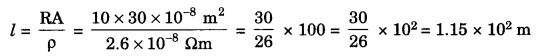Question 6.
Two coils of resistance R1 = 3Ω and R2 = 9Ω are connected in series across a battery of potential difference 14 V. Draw the circuit diagram. Find the electrical energy consumed in 1 min in each resistance.
Given: R1 = 3Ω, R2 = 9Ω
Rs = R1 + R2 = 9 + 3 = 12 ΩNow, I = $$\frac{V}{R}=\frac{14}{12}$$ = 1.167 Amp. [I in series remains constant.]
Electric energy consumed in R1
H1 = I2R1t = (1.167)2 × 3 × 60 = 245.14 J
Electric energy consumed in R2
H2 = I2R2t = 735.42J

Question 7.
State the relation between work, charge and potential difference for an electric circuit.
Calculate the potential difference between the two terminals of a battery if 100 joules of work is required to transfer 20 coulombs of charge from one terminal of the battery to the other.
V = $$\frac{W}{Q}$$
Here,
V = Potential difference,
W = Work done,
Q = Electric charge
W = 100 J
Q = 20 C
V = $$\frac{W}{Q}=\frac{100}{20}$$ = 5V

Question 8.
What is an electric circuit? Distinguish between an open and a closed circuit.
Electric circuit: A continuous and closed path of electric current is called an electric circuit.
Open circuit: A discontinuous circuit through which no current can flow.
Closed circuit: A circuit without interruption, providing a continuous path through which a current can flow.

### Extra Questions for Class 10 Science Chapter 12 Short Answer Type II

Question 1.
With the help of a diagram, derive the formula for the equivalent resistance of three resistances connected in series.
(i) If a number of resistances are connected in such a way that the same current flows through each resistance, then the arrangement is called ‘Resistances in Series’.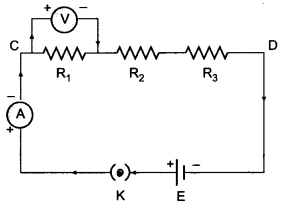(ii) Let R1, R2 and R3 be three resistances connected in a series combination and let R be their equivalent resistance.
Let V1, V2 and V3 be the potential difference across the resistances R1, R2 and R3 respectively. Let ‘V’ be the potential differences across the combination. Let ‘I’ be the current flowing through each resistance.

(iii) According to Ohm’s law,
V = IR
Hence, V1 = IR1; V2 = IR2; V3 = IR3
(iv) For series combination of resistances,
V = V1 + V2 + V3
IRs = IR1 + IR2 + IR3
IRs = I (R1 + R2 + R3)
Rs = R1 + R2 + R3
Hence, the equivalent resistance in series (Rs) is equal to the sum of the individual resistances.

Question 2.
With the help of a diagram, derive the formula for the equivalent resistance of three resistances connected in parallel.
1. If a number of resistances are connected between two common points in such a way that the potential difference across each resistance is same, then the arrangement is called ‘Resistances in Parallel’.

2. Let R1, R2 and R3 be the three resistances connected in parallel combination between points C and D and let Rp be their equivalent resistance.
Let I1, I2 and I3 be the currents flowing through resistances R1, R2 and R3 respectively.
Let I be the current flowing through the circuit and V be the potential difference of the cell.

3. According to Ohm’s law.
I = $$\frac{V}{R}$$
Therefore,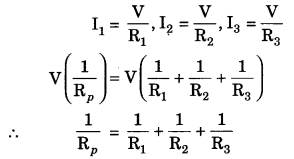Therefore, the reciprocal of the equivalent resistances in parallel combination is equal to the sum of the reciprocals of the individual resistances.

Question 3.
What is the better way of connecting lights and other electrical appliances in domestic wiring? Why?
The better way of connecting lights and other electrical appliances in domestic wiring is parallel connection because of the following advantages:

• In parallel circuit, if one appliance stops working due to some defect, then all other appliances keep working normally.
• In parallel circuit, each electrical appliance has its own switch due to which it can be turned on or off, without affecting other appliances.
• In parallel circuit, each electrical appliance gets the same voltage (220 V) as that of the power supply line.
• In parallel circuit, the overall resistance of the domestic circuit is reduced due to which the current from the power supply is high.

Question 4.
An electric lamp, whose resistance is 20 Ω, and a conductor of 4 Ω resistance are connected to aft V battery (Fig.).
Calculate (a) the total resistance of the circuit, (b) the current through the circuit, and (c) the potential difference across the electric conductor.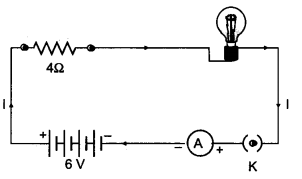(a) Resistance of electric lamp, R1 = 20 W
Resistance of series conductor, R2 = 4 W
Total resistance in the circuit,
Rs = R1 + R2 = 20 Ω + 4 Ω = 24 Ω.

(b) Total potential difference, V = 6 V
By Ohm’s law, the current through the circuit is(c) Potential difference across the electric lamp,
V1 = IR1 = 0.25 A × 20 Ω = 5 V.
Potential difference across the conductor is
V2 = IR2 = 0.25 A × 4 Ω = 1 V.

Question 5.
A wire has a resistance of 10Ω. It is melted and drawn into a wire of half of its length. Calculate the resistance of the new wire. What is the percentage change in its resistance?
Given: R1 = 10 Ω, l2 = $$\frac{l_{1}}{2}$$
To find: (a) R2
(b) Percentage change in the resistance (ΔR%).
If volume of the wire remains same in both the cases.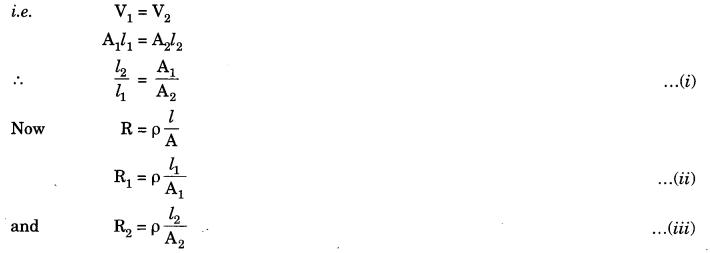Dividing eq. (iii) by (ii), we getQuestion 6.
If, in Figure R1 = 10 ohms, R2 = 40 ohms, R3, = 30 ohms, R4 = 20 ohms, Rg = 60 ohms and a 12 volt battery is connected to the arrangement, calculate: (a) the total resistance and (b) the total current flowing in the circuit.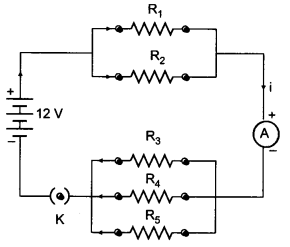(a) Let R’ be the equivalent resistance of R1 and R2. Then,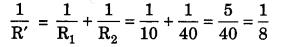R’ = 8Ω
Let R” be the equivalent resistance of R3, R4 and R5. Then,R” = 10 Ω
Total Resistance, R = R’ + R” = 8 + 10 = 18 Ω

(b) Current,Question 7.
Two lamps, one rated 60 W at 220 V and other 40 W a 220 V, are connected in parallel to an electric supply at 220 V. [CBSE 2016]
(a) Draw the circuit diagram to show the connections.
(b) Calculate the current drawn from the electric supply.
(c) Calculate the total energy consumed by the two lamp together when they operate for one hour.
(a) The required circuit diagram is shown below: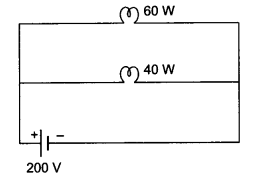(b) Total power of the two lamps = 60 + 40 = 100 W
Applied Voltage, V = 220 V
Current drawn from the electric supply,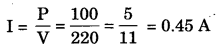(c) Total energy consumed by the lamp in one hour = 60W × 1h + 40W × 1h = 100 Wh = 0.1 kWh.

Question 8.
A household uses the following electric appliances:
(i) Refrigerator of rating 400 W for ten hours each day.
(ii) Two electric fans of rating 80 W each for twelve hours each day.
(iii) Six electric tubes of rating 18 W each for 6 hours each day.
Calculate the electricity bill of the household for the month of June if the cost per unit of electric energy is ₹ 3.00.
Energy consumed by refrigerator of rating 400 W for ten hours each day
= P × t = 400 W × 10 Aw = $$\frac{400}{1000}$$ kW × 10 h = 4.0 kWh
Energy consumed by two electric fans of rating 80 W each for twelve hours each day
= 2 × P × t = 2 × 80W × 12h = $$\frac{160}{1000}$$ kW × 12 h = 1.92 kWh
Energy consumed by six electric tubes of rating 18 W each for 6 hours each day
= 6 × P × t = 6 × 18W × 6h = $$\frac{108}{1000}$$ W × 6 h = 0.648 kWh
Total energy consumed in the month of June (30 days)
= (4.0 + 1.92 + 0.648) × 30 kWh = 6.568 × 30 = 197.04 kWh
Electricity bill for the month of June
= ₹ 197.04 × 3 = ₹ 591.12 = ₹ 591 (approx.)

### Extra Questions for Class 10 Science Chapter 12 Long Answer Type

Question 1.
(a) Two resistors R1 and R2 may form (i) a series combination or (ii) a parallel combination, and the combination may be connected to a battery of six volts. In which combination will the potential difference across R1 and across R2 be the same and in which combination will the current through R1 and through R2 be the same?
(b) For the circuit, shown in this diagram,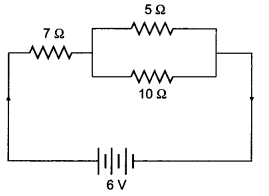Calculate
(i) the resultant resistance
(ii) the total current
(iii) the voltage across 7 Ω resistor
(a)In series combination, the current through R1 and R2 is same.
In parallel combination, the potential difference across R1 and R2 is same.

(b) (i) Here 5Ω and 10Ω resistors are connected in parallel.Now circuit becomes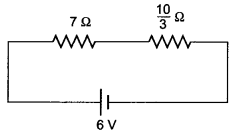Equivalent resistance,
Req = $$\frac{10}{3}$$Ω + 7Ω = $$\frac{31}{3}$$Ω = 10.33Ω
(ii)(iii) Voltage across 7Ω resistor
V = IR ⇒ V = $$\frac{18}{31}$$ × 7 = 4.06A

Question 2.
(a) List the factors on which the resistance of a conductor depends.
(b) A 4 kW heater is connected to a 220 V source of power. Calculate
(i) the electric current passing through the heater.
(ii) the resistance of the heater.
(iii) the electric energy consumed in 2 hour use of the heater.
(a) Resistance of a conductor depends on
(i) length of conductor (l)
(ii) Area of cross-section (A)
(iii) Resistivity of material (ρ)
Relation of resistance is given by, R = ρ$$\frac{l}{A}$$

(b) Given, P = 4 kW = 4000 W and V = 220 V
(i) P = VI or I = $$\frac{P}{A}$$
Hence, I = $$\frac{4000}{220}$$ = 18.18 A

(ii) V = IR or R = $$\frac{V}{I}$$
R = $$\frac{220}{18.18}$$ = 12.1Ω

(iii) Energy consumed, E = P × t
E = 4000 W × 2h = 8000Wh = 8 kWh = 8 unit.

Question 3.
(a) State Ohm’s law.
(b) Give the circuit diagram to establish relation between potential difference (V) and current (I) through a given wire.
(c) Draw a graph between V and I.
(a) Ohm’s law:
According to Ohm’s law, the potential difference, v, across the ends of a given metallic wire in an electric circuit is directly proportional to the current flowing through it, provided its temperature remains same.
V ∝ I ⇒ $$\frac{V}{I}$$ = constant
$$\frac{V}{I}$$ = R (R = constant) ⇒ V = IR
Here, R is constant for a given metallic wire at a given temperature and is called resistance.
V-I graph of Ohm’s law and its experimental setup:

(b) Circuit diagram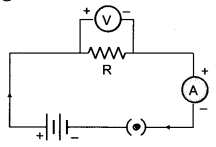(c) Variation of current with potential difference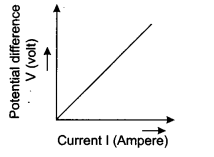### Electricity HOTS Questions With Answers

Question 1.
Two students perform experiments on series and parallel combinations of two given resistors R1 and R2 and plot the following V-I graphs.Which of the graphs is (are) correctly labeled in terms of the words ‘Series and parallel’? justify your answer.
In case of series combination, the effective resistance = R1 + R2 is more, hence slope of V – I graph will be more. It is otherwise in case of I – V graph. So, series and parallel are correctly marked in graph (ii).

Question 2.
You are given three resistors of 10 Ω, 10 Ω, 20 Ω to a battery of emf 2.5 V, a key, an ammeter and a voltmeter. Draw a circuit diagram showing the correct connections of given components such that the voltmeter gives a reading of 2.0 V.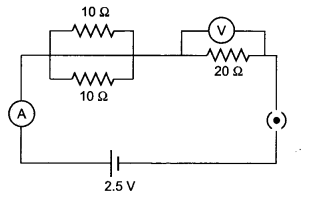Question 3.
The electrical resistivity of few material is given below in ohm-metre. Which of these materials can be used for making elements of a heating device.
A 6.84 × 10-8 Ωm
B 1.60 × 10-8 Ωm
C 1.00 × 10-4 Ωm
D 2.50 × 1012 Ωm
E 4.40 × 10-5 Ωm
F 2.30 × 1017 Ωm
A material having highest value of resistivity is used for making element of heating devices; therefore, material C will be used. D and F are insulators since they have very high values of resistivity.

Question 4.
Two electric bulbs A and B are marked 220 V, 60 W and 220 V, 100 W respectively. Which one of the two has greater resistance?
The resistance of a bulb is given by the expression R = V2/P. For the voltage, the bulb having a smaller power has more resistance. Therefore, the 60W, 220 V bulb has a greater resistance.

Question 5.
A potential difference V is applied across a conductor of length l and diameter D. How is the resistance R of the conductor affected, when (i) V is halved (ii) l is halved and (iii) D is doubled. Justify your answer in each case.
The table below gives the variation:Question 6.
The applied potential difference across a given resistor is altered so that the heat produced per second increases by a factor of 16. By what factor the applied potential difference change.
The heat produced across a resistor is given by R = V2/P. It is proportional to the square of potential.
Therefore, if the heat becomes 16 times the voltage must have been increased 4 times.

Question 7.
The I – V graphs of two resistors, and their series combination, are shown below. Which one of these graphs represents the series combination of the other two? Give reasons for your answer.Draw a vertical line from any point on the V axis such as to cut the graphs 1, 2 and 3 at points P, Q and R as shown in the graph below.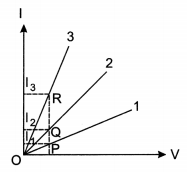For the same potential, the current in each is different (I1 < I2 < I3). Hence $$\frac{V}{I_{1}}$$ will be greatest for graph 1.
Therefore, graph 1 represents the series combination of the other two resistances.

Question 8.
The values of current I flowing in a given resistor for the corresponding values of potential difference V across the resistor are given below:

 I (amperes) 0.5 1 2 3 4 V (volts) 1.6 3.4 6.7 10.2 13.2

Plot a graph between V and I and calculate the resistance of the resistor.
The graph between V and I for the given data is shown below: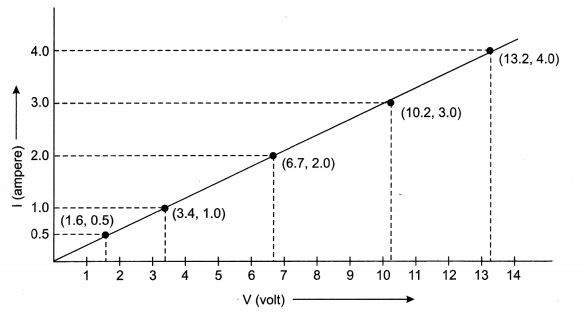Resistance of the resistor,Question 9.
A piece of wire having resistance ‘R’ is cut into four equal parts.
(a) How does the resistance of each part compare with the original resistance?
(b) If the four parts are placed in parallel, how will be the resistance of the combination compare with the resistance of the original wire?
(a) As R ∝ l, when the wire is cut into four equal pieces, the resistance of each part is $$\frac{R}{4}$$
(b) When they are connected in parallel.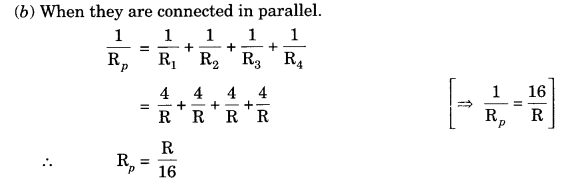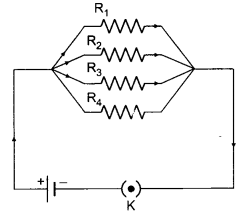Question 10.
Two resistance when connected in parallel give resultant value of 2 Ω. When connected in series, the value becomes 9 Ω. Calculate the value of each resistance.
Let R1 and R2 be the two resistances,
R1 + R2 = 9Ω  …….(1)
When connected in parallelNow (a – b)2 = (a + b)2 – 4ab
(R1 – R2)2 = (R1 + R2)2 – 4 R1R2 = 9 × 9 – 4 × 18
(R1 – R2)2 = 81 – 72 = 9 or R1 – R2 = 3
∴ R1 – R2 = 3  ………(2)
Solving eq. (i) and (ii), we gets
R1 + R2 = 9 or R1 – R2 = 3
2R1 = 12
∴ R1 = 6Ω
Putting the value of R1 in eq. (i), we get
R1 + R2 = 9 or R2 = 9 – R1 = 9 – 6
R2 = 3Ω

Question 11.
If length of a resistance wire become half and cross-section area becomes twice, then find the relation between old resistance and new resistance.Dividing (ii) by (i), we get R’ = $$\frac{R}{4}$$
Resistance becomes one fourth.

### Extra Questions for Class 10 Science Chapter 12 Value Based Questions

Question 1.
Raman always switched off lights, fans and electric gadgets when not in use. Her electricity bill had cut down to half.
(a) What is commercial unit of electric energy?
(b) Suggest any two methods of saving electricity,
(c) What values does Raman show?